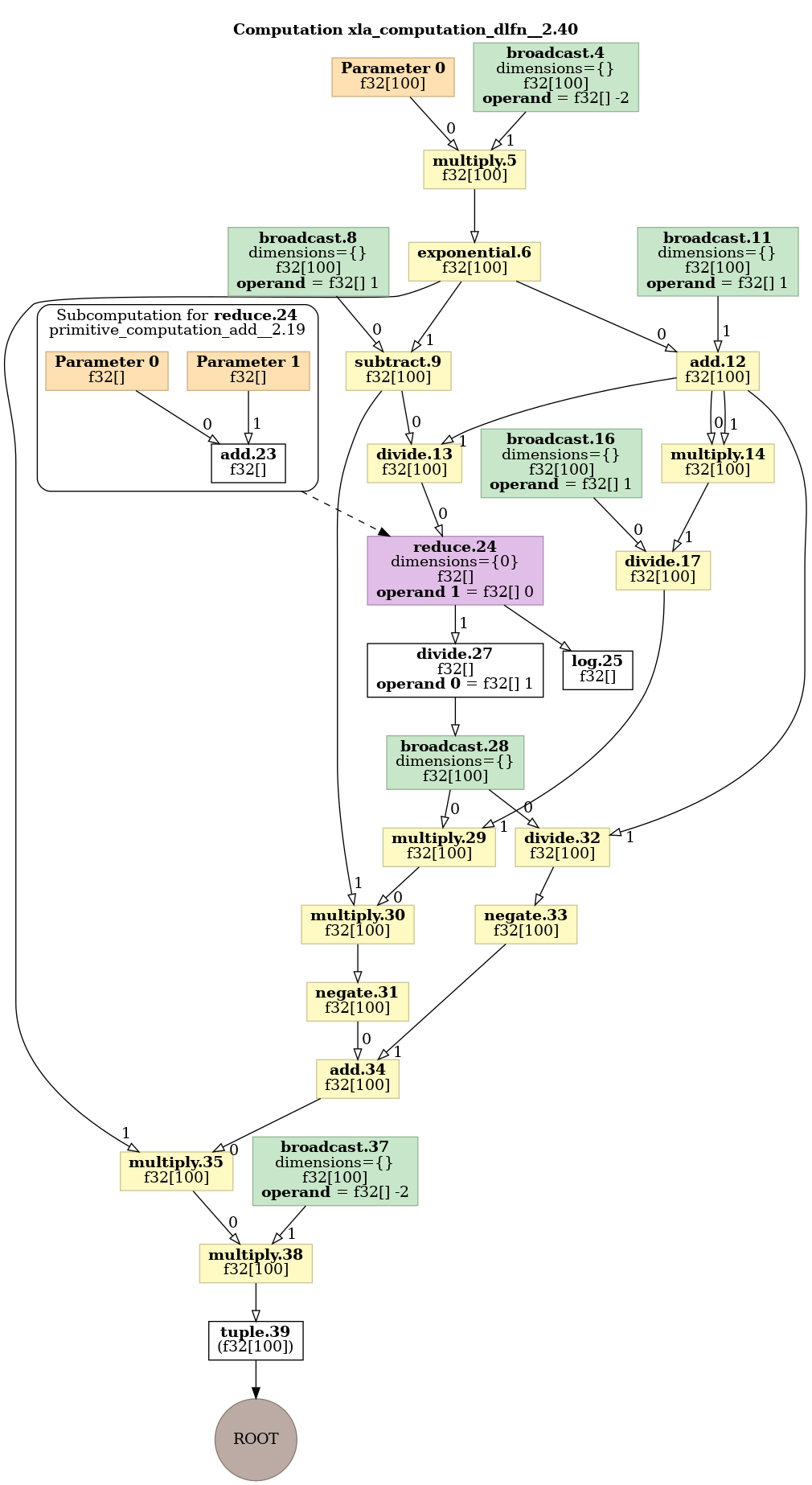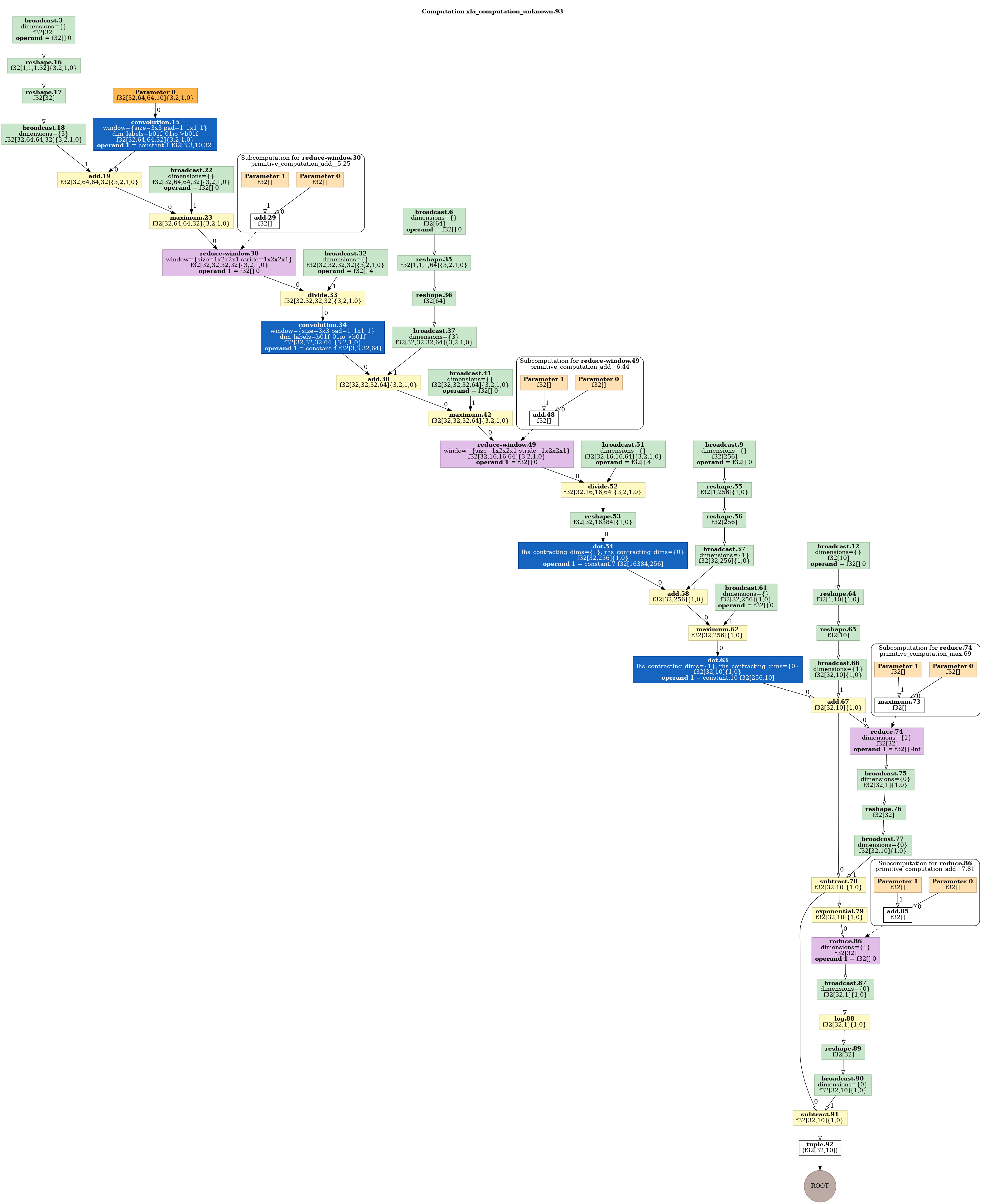I’ve written while ago about visualising the computational graph of a JAX program here. Jax has evolved since then, so here is an update for the current (as of time of writing) version of Jax (0.3.1).

## Export the HLO Intermediate Representation (IR)

The main change is that the graph is produced from an jax.xla_computation object. The other change is that instead of specifying the input shape I am supplying an example array (numpy.ones(100)).

Here is the updated program:


import jax

def tanh(x):
y = numpy.exp(-2.0 * x)
return (1.0 - y) / (1.0 + y)

def lfn(x):
return numpy.log(tanh(x).sum())

def dlfn(x):

z=jax.xla_computation(dlfn)(numpy.ones(100))

with open("t.txt", "w") as f:
f.write(z.as_hlo_text())


This will create a text file t.txt with a text-based HLO.

## Visualise

The simplest way of visualising is to dump as a dot graph and run dot:

with open("t.dot", "w") as f:
f.write(z.as_hlo_dot_graph())

dot t.dot  -Tpng > t.png


This produces the following image:## Visualise a neural network

The same basic approach can be used to visualise a flax neural network, e.g.,:

import functools
import flax.linen as nn

class CNN(nn.Module):
@nn.compact
def __call__(self, x):
x = nn.Conv(features=32, kernel_size=(3, 3))(x)
x = nn.relu(x)
x = nn.avg_pool(x, window_shape=(2, 2), strides=(2, 2))
x = nn.Conv(features=64, kernel_size=(3, 3))(x)
x = nn.relu(x)
x = nn.avg_pool(x, window_shape=(2, 2), strides=(2, 2))
x = x.reshape((x.shape, -1))  # flatten
x = nn.Dense(features=256)(x)
x = nn.relu(x)
x = nn.Dense(features=10)(x)
x = nn.log_softmax(x)
return x

model = CNN()
batch = numpy.ones((32, 64, 64, 10))  # (N, H, W, C) format
variables = model.init(jax.random.PRNGKey(0), batch)

f=functools.partial(model.apply, variables)
z=jax.xla_computation(f)(batch)

with open("t2.dot", "w") as f:
f.write(z.as_hlo_dot_graph())


This produces the following image: